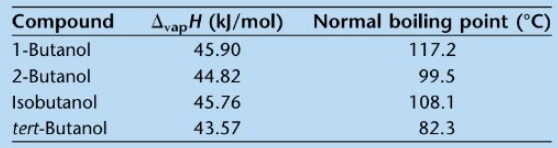# Problem: Four alcohols have the formula C4H9OH:1-butanol, 2-butanol (or sec-butanol), isobutanol (or 2-methyl-1-propanol), and tert-butanol (or 2-methy;-2-propanol).They are examples of isomers, or compounds that have the same molecular formula but different molecular structures. The below table gives data on the isomers:Using the Clausius-Clapeyron equation, rank the isomers of butanol in order of decreasing vapor pressure at 25 °C. Does the ranking agree with any conventional wisdom based on the ΔvapH Values or the normal boiling points?

###### FREE Expert Solution
91% (233 ratings)
###### Problem Details

Four alcohols have the formula C4H9OH:

1-butanol, 2-butanol (or sec-butanol), isobutanol (or 2-methyl-1-propanol), and tert-butanol (or 2-methy;-2-propanol).

They are examples of isomers, or compounds that have the same molecular formula but different molecular structures. The below table gives data on the isomers:

Using the Clausius-Clapeyron equation, rank the isomers of butanol in order of decreasing vapor pressure at 25 °C. Does the ranking agree with any conventional wisdom based on the ΔvapH Values or the normal boiling points?What scientific concept do you need to know in order to solve this problem?

Our tutors have indicated that to solve this problem you will need to apply the Clausius-Clapeyron Equation concept. You can view video lessons to learn Clausius-Clapeyron Equation. Or if you need more Clausius-Clapeyron Equation practice, you can also practice Clausius-Clapeyron Equation practice problems.

What is the difficulty of this problem?

Our tutors rated the difficulty ofFour alcohols have the formula C4H9OH:1-butanol, 2-butanol (...as high difficulty.

How long does this problem take to solve?

Our expert Chemistry tutor, Dasha took 16 minutes and 51 seconds to solve this problem. You can follow their steps in the video explanation above.

What professor is this problem relevant for?

Based on our data, we think this problem is relevant for Professor McCarren's class at UIUC.